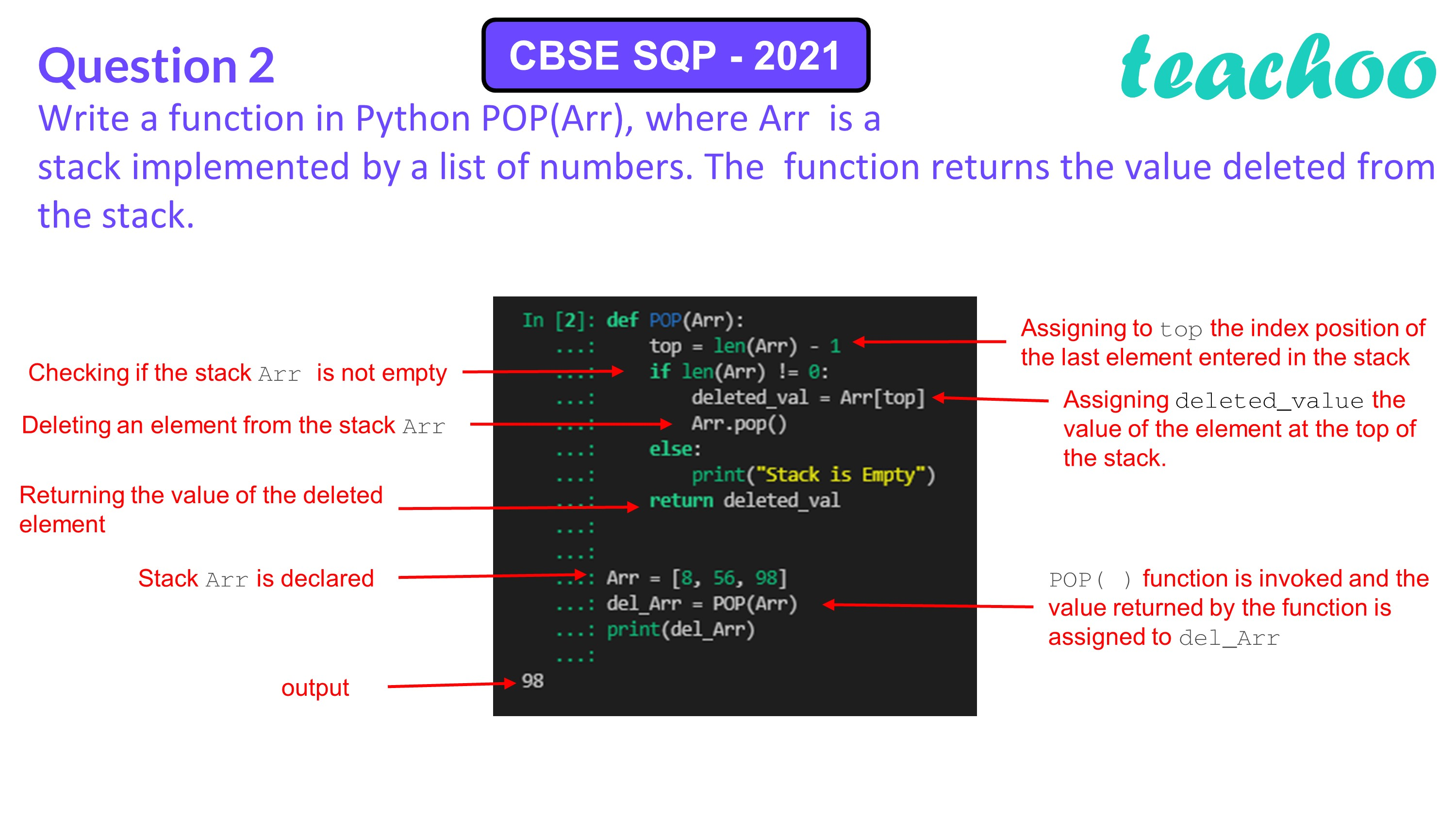Past Year - 3 Mark Questions

Computer Science - Class 12
Chapter 3 - Stacks

## Write a function in Python POP(Arr), where Arr is a stack implemented by a list of numbers. The function returns the value deleted from the stack.

``` def POP(Arr): ```

``` top=len(Arr)-1 ```

``` if len(Arr)!=0: ```

``` deleted_val=Arr[top] ```

``` Arr.pop() ```

``` else: ```

``` print("Stack is Empty") ```

``` return deleted_val ```Learn in your speed, with individual attention - Teachoo Maths 1-on-1 Class

### Transcript

Write a function in Python POP(Arr), where Arr is a stack implemented by a list of numbers. The function returns the value deleted from the stack. Answer: def POP(Arr): top=len(Arr)-1 if len(Arr)!=0: deleted_val=Arr[top] Arr.pop() else: print("Stack is Empty") return deleted_val